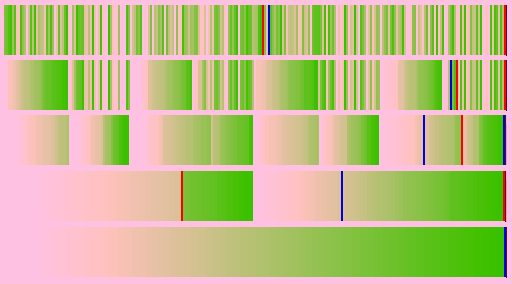Simulates and represents some of the traditional sequential sorting algorithms. Developed some contributed algorithms by merging two or more algorithms, or by applying parallel programming concepts by dividing the sorting task into small tasks and distributing them between available processors equally without any conflict between them to get better results.

The base class of all sorting algorithm has set of methods that help counting the comparisons and swaps operations, in addition to calculate the time complexity of each step by adding time unit(s) to the algorithm complexity. JFrame interface is used to clarify and demonstrate each algorithm sorting mechanism.

• Use JFrame to simulate sorts algorithms.
• Implement a multi-threaded algorithm, that calculate the time and space complexity.